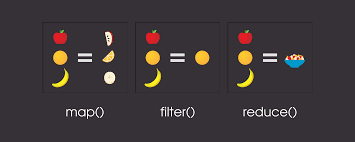{{announcement.body}}
{{announcement.title}}

# Map and Filter Function in Python

DZone 's Guide to

# Map and Filter Function in Python

### In this article, learn about some of the most used python function maps in machine learning.

· AI Zone ·
Free Resource

Comment (0)

Save
{{ articles.views | formatCount}} ViewsMachine learning is no doubt the most trending topic right now, and python is the most used versatile language in machine learning.

In this article, I’ll tell you about some of the most used python function maps in machine learning.

## Map

The map function is used to manipulate a list and get an iterable object out of it. It does not return a list, it returns an object of type map.

The map function takes in a function as a first parameter and list as a second parameter, and then it will iterate over every item in the list and apply that passed function to it. Then it will add it to the new collection and return that collection in the end.

In the example below, we have a function get_cube, which will take a number and return the cube of it.

Then, we have a list =[1,2,3,4,5], and we will apply the map function and pass the function (get_cube ) and the list to it.

In the end, we are printing the type of value return from the map function and the actual value in the object(updated_list), and then we are iterating through all the elements in the new list.

``def get_cube(num):    return num**3``
``lst = [1,2,3,4,5]updated_lst = map(get_cube,lst)print(type(updated_lst))print(updated_lst)``
``for i in updated_lst:    print(i,end=",")``

The output will be:

``<class 'map'><map object at 0x000002C760BC7278>1,8,27,64,125,``
Note: The code below will have the same functionality as the code above. This is a more concise way to write python code.
``lst = [1,2,3,4,5]updated_lst = map(lambda num:num**3 ,lst)print(type(updated_lst))print(updated_lst)print([i for i in updated_lst],end=",")``
The result of map function can be achieved by list comprehension also, but the map is faster.

The new iterable object returned from the map function can be easily converted to list set or tuple as follows:

``lstNew = list(updated_lst)print(lstNew)``
``lstNew = set(updated_lst)print(lstNew)``
``lstNew = tuple(updated_lst)print(lstNew)``

## Filter Function

The filters method filters the given iterable with the help of a function that tests each element in the iterable to be true or not.

The filter function will return an iterable object of the filter class.

Let’s say if we have a list from 0 to 100:

``lst = list(range(50))print([i for i in lst],end=",")``
``#output[0, 1, 2, 3, 4, 5, 6, 7, 8, 9, 10, 11, 12, 13, 14, 15, 16, 17, 18, 19, 20, 21, 22, 23, 24, 25, 26, 27, 28, 29, 30, 31, 32, 33, 34, 35, 36, 37, 38, 39, 40, 41, 42, 43, 44, 45, 46, 47, 48, 49]``

Now, suppose we want to take only the odd numbers from this list, or more clearly, we want to filter all the odd numbers from this list.

We first need the condition of how we will identify whether the number is odd or not.

Let’s create a function odd_func, which will return true or false based on if the number is odd or not.

The function passed to filter function should return boolean:

``def is_odd(num):     return num%2!=0``

To use it with a list for filter, we will pass it as an argument in the filter function.

``filter_lst = filter(is_odd,lst)print(type(filter_lst))``
``#output<class 'filter'>``

We can convert this filter obj to list by simply passing it to list function.

``filter_lst = list(filter_lst)``

## Filter Function With Lambda Function

Filter function takes the function, which returns true or false, so they are very short functions, hence we can use lambdas to make it short and sweet. Let's see the lambda version of filter functions:

``lst = list(range(50))filter_lst = filter(lambda x:x%2 !=0,lst)print(type(filter_lst))``

Thanks for taking the time to read this, and let me know if you have any questions in the comments below.

Topics:
artificial intelligence, functions, lambda functions, machine learning, python, python functions

Comment (0)

Save
{{ articles.views | formatCount}} Views

Opinions expressed by DZone contributors are their own.